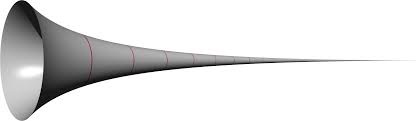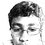# The Gabriel HornThis paradox is not original

Consider a horn with an open side, which becomes thinner and thinner as the horn's length approaches infinity

Statement:

Is the amount of paint is needed to cover the inside of the horn finite or infinite?

Possible Solutions:

Since the inside of the horn has infinite surface area, and the paint must cover the infinite surface area, the amount of paint is infinite

However, the horn has finite volume as it closes at infinity. Thus, if we took a can of paint of the same volume as the horn, and poured it into the horn, the paint would cover the entire inner surface area. Thus, the amount of paint is finite

Thus, arises the paradox!Note by Nanayaranaraknas Vahdam
6 years, 8 months ago

This discussion board is a place to discuss our Daily Challenges and the math and science related to those challenges. Explanations are more than just a solution — they should explain the steps and thinking strategies that you used to obtain the solution. Comments should further the discussion of math and science.

When posting on Brilliant:

• Use the emojis to react to an explanation, whether you're congratulating a job well done , or just really confused .
• Ask specific questions about the challenge or the steps in somebody's explanation. Well-posed questions can add a lot to the discussion, but posting "I don't understand!" doesn't help anyone.
• Try to contribute something new to the discussion, whether it is an extension, generalization or other idea related to the challenge.
• Stay on topic — we're all here to learn more about math and science, not to hear about your favorite get-rich-quick scheme or current world events.

MarkdownAppears as
*italics* or _italics_ italics
**bold** or __bold__ bold
- bulleted- list
• bulleted
• list
1. numbered2. list
1. numbered
2. list
Note: you must add a full line of space before and after lists for them to show up correctly
paragraph 1paragraph 2

paragraph 1

paragraph 2

[example link](https://brilliant.org)example link
> This is a quote
This is a quote
    # I indented these lines
# 4 spaces, and now they show
# up as a code block.

print "hello world"
# I indented these lines
# 4 spaces, and now they show
# up as a code block.

print "hello world"
MathAppears as
Remember to wrap math in $$ ... $$ or $ ... $ to ensure proper formatting.
2 \times 3 $2 \times 3$
2^{34} $2^{34}$
a_{i-1} $a_{i-1}$
\frac{2}{3} $\frac{2}{3}$
\sqrt{2} $\sqrt{2}$
\sum_{i=1}^3 $\sum_{i=1}^3$
\sin \theta $\sin \theta$
\boxed{123} $\boxed{123}$

## Comments

Sort by:

Top Newest

Not any surface of revolution that looks like a horn has this property, but one that is based on the function $y=1/x$ does, and is the one called "Gabriel's Horn". For $1\le x\le \infty$, it has finite volume but infinite surface area. But because the thickness of the paint inside the horn cannot exceed the radius, when we compute the volume of the paint assuming a thickness of $1/x$ as $x\rightarrow \infty$, the total volume of the paint does drop down to zero, not infinity, and thus the paradox is resolved.

- 6 years, 8 months ago

Log in to reply

I doubt if that is a paradox. A finite volume of paint can always have an infinite area and can be used to paint an infinite area because the paint is infinitesimally thin.

Moreover, the finite paint can paint an infinite area because an object with finite volume can have infinte surface area just like the horn.

- 6 years, 8 months ago

Log in to reply

Not even paradox

- 6 years, 8 months ago

Log in to reply

Any one explain how this gabriel horn got finite volume physically not mathematically. What is its physical interpretation?

- 5 years, 8 months ago

Log in to reply

Just looking back at this note now - what's up with my explanation? Something wrong??

- 5 years, 8 months ago

Log in to reply

Hey, what up man?

This would be a true paradox if it can be shown that even if the paint is applied in an ever thinner layer as one goes deeper into the trumpet, the volumetric amount of paint becomes infinite. So, propose a function T(x), where x is axial distance from the entrance of horn, that is the thickness of the paint, less than y(x), which is the function of the curve of the horn, such that the volume becomes infinite. You can pick any T(x) and y(x), provided both are monotonic.

- 5 years, 8 months ago

Log in to reply

Did I just hear you say "what up man?" ...

...

...

...

HELL YEAH!!

What's up? A lot. It's called COLLEGE. What a DISASTER. I spend days and nights writing lab reports!!! Oh there's a lot to tell. I'll tell you more when I get home (or probably tomorrow - 3 lab reports to write!) - right now I've challenged a dude to a piano contest - gonna wreck him ;)

L8rz!

- 5 years, 8 months ago

Log in to reply

You're gonna bang some wild Franz Liszt pieces?

- 5 years, 8 months ago

Log in to reply

Oh HEEELL naww. If you're talking about stuff like this, no way. But I'm not too noob either. And we had to reschedule it anyway. I'll make a "perfect" recording and upload it to the loneliest corner on the internet - and share it with you ;) Can't guarantee my face will be in it! But you'll get to see it too in some other things I've planned that I shall discuss probably... tomorrow - no worries I'm no Quasimodo lol

Physics III quiz coming up! Gotta read teh whole chapter before that. Gonna get crackin

- 5 years, 8 months ago

Log in to reply

In the real world, it's obvious that such an arrangement would not occur. No paradox here.

But as far as math is concerned, then there's still no paradox: the paint is only concerned with filling the volume, NOT covering the surface area; those are two different things. The space inside the horn is the space that is inside the horn only, NOT including the space in the composition of the horn itself. Even though mathematically this horn may have a one dimensional depth (or, no depth, as some people like to say (inaccurately)) - so, it's a two-dimensional entity - it still contributes to the amount the paint has to fill. Oh, and for the record, surface area does contribute to the volume. Not going to explain this now, but it does.

So, in conclusion, if the volume is finite, the paint fills it completely, regardless of whether the surface area is finite or infinite.

Another look:

Consider the amount of Area that needs to be filled vs. the amount of Volume that needs to be filled.

From integral calculus (or just a solid understanding of how areas/volumes are formed), we know that for each unit of depth of the horn, the paint has to fill $2\pi r$ surface area units and $\pi r^2$ volume units (note - PER UNIT implies that an additional unit of length is incorporated - thus giving the third dimension to $r^2$ and 2nd to $r$). And as we may know, the volume unit shrinks much quicker than the area unit - that is, when the radius shrinks lower than $1$.

Ex.:

$r=0.5$

$A= 2\pi \cdot 0.5 = \pi$

$V=\pi \cdot (0.5)^2=0.25 \pi$.

Ratio: $\frac{1}{4}$

$r=0.1$

$A=0.2 \pi$

$V= 0.01 \pi$

Ratio: $\frac{1}{50}$

...

So you can pretty much draw a sums comparison between $p$ values - harmonics ($r$) diverge, whereas $r^2$ converges. And since filling the volume with paint can alternatively be thought of as the volume of the horn itself, then since that is finite, so is paint. And as we saw above, a finite volume can cover an infinite surface area.

Cheers

- 6 years, 8 months ago

Log in to reply

×

Problem Loading...

Note Loading...

Set Loading...